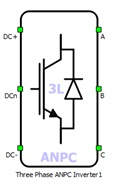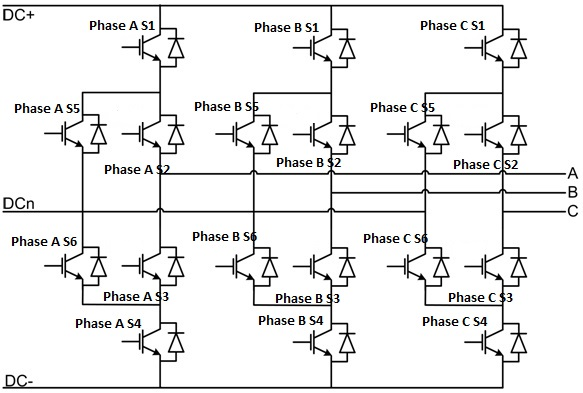# Three Phase ANPC Inverter

Description of the Three Phase ANPC Inverter component in Schematic Editor

A block diagram and input parameters for an inverter-rectifier block are given in Table 1.

Table 1. Three-phase three-level ANPC inverter block in the Schematic Editor core library
component component dialog window component parametersThree phase three level ANPC inverter• General
• Control(Digital inputs, Model)
• Phase A S1 (1..32)
• Phase A S2 (1..32)
• Phase A S3 (1..32)
• Phase A S4 (1..32)
• Phase A S5 (1..32)
• Phase A S6 (1..32)
• Phase B S1 (1..32)
• Phase B S2 (1..32)
• Phase B S3 (1..32)
• Phase B S4 (1..32)
• Phase B S5 (1..32)
• Phase B S6 (1..32)
• Phase C S1 (1..32)
• Phase C S2 (1..32)
• Phase C S3 (1..32)
• Phase C S4 (1..32)
• Phase C S5 (1..32)
• Phase C S6 (1..32)
• gate_logic (active high, active low)
• Gate control enabling (enables usage of external digital signal to enable the switching)
• Sen (digital input selection for PWM enable)
Weight = 3

A schematic block diagram of the inverter switching block is given in Figure 1 with corresponding switch arrangement and naming.

Digital inputs, when selected as the Control parameter, enables you to assign gate drive inputs to any of the digital input pins (from 1 to 32). For example, if Phase A S1 is assigned to 1, the digital input pin 1 will be routed to the Phase A S1 switch gate drive. In addition, the gate_logic parameter selects either active high(High-level input voltage VIH turns on the switch), or active low (Low-level input voltage VIL turns on the switch). The gate drive logic depends on your external controller design.

Model, when selected as the Control parameter, enables you to set the IGBTs' gate drive signals directly from signal processing model. The input pin gates appears on the component. It requires a vector input of eighteen gate drive signals in the following order: [Phase A S1, Phase A S2, … , Phase B S1, Phase B S2, … , Phase C S1, Phase C S2, ...]. When controlled from the model, logic is always set to active high.

Gate control enabling, when checked, enables use of an external PWM enabling digital signal.Figure 1. A schematic block diagram of a three-phase three-level ANPC inverter/rectifier block diagram with corresponding switch naming

## Losses calculation

When the Losses calculation property is enabled, the component will calculate switching and conduction power losses for all switching elements (IGBTs and Diodes or MOSFETs). In the case of MOSFET switching elements, the diode characteristic represents the internal MOSFET body diode. Switching power losses are calculated as a function of current, voltage, and temperature using 3D lookup tables. Also, 2D input for losses is supported. When a 2D losses table is inserted, it assumes only current (I) and temperature dependence. Conduction power losses can be defined as a function of current and temperature using Vt and Vd lookup tables (LUTs). These LUTs can be 1D or 2D tables. If the LUT is a 1D table, forward voltage drop depends only on the current, but if LUT is a 2D table, forward voltage drop dependence on the junction temperature is also considered. In the MOSFET case under reverse current conduction, a current sharing calculation between the MOSFET channel and the internal body diode is performed. Import options and an explanation how to correctly fill all necessary power losses parameters is described in the Importing power losses data section.

In the case of the IGBT switch type, input/output terminals for power losses receive/generate vectors of 36 elements (indexes 0-35, where every index in the vector represent one switching element). The first 12 elements (base index 0) are related to the switching elements in phase A, the second 12 elements (starting from base index 12) are related to the switching elements in phase B and the last 12 elements (with the base index 24) are related to the switching elements in phase C.

For the MOSFET switch type, for all Losses groups, input/output terminals for power losses receive/generate vectors of 18 elements (every index in the vector represent one switching element). The first 6 elements (indexes 0-5) are related to the switching elements in phase A, the second 6 elements (indexes 6-11) are related to the switching elements in phase B, and the last 6 elements (indexes 12-17) are related to the switching elements in phase C. If different switch types are used for the Losses groups, input/output terminals for power losses receive/generate vectors with the same ordering logic as previously described. Switching element ordering and group definition for each phase are described in the ANPC Leg Losses calculation section.

All switches are distributed in three groups, and for each group, different power loss parameters can be specified (Phase A S1, Phase A S4, Phase B S1, Phase B S4, Phase C S1, and Phase C S4 are in group 1; Phase A S2, Phase A S3, Phase B S2, Phase B S3, Phase C S2, and Phase C S3 are in group 2; Phase A S5, Phase A S6, Phase B S5, Phase B S6, Phase C S5, and Phase C S6 are in group 3).

Available component properties are:

• Losses groups - Switching elements group
• Current values - Switching elements current axis [A]

• Voltage values - Switching elements voltage axis [V]

• Temp values - Switching elements temperature axis [°C]

• Vt table - Switch forward voltage drop, f(I,T) [V]

• Vd table - Diode forward voltage drop, f(I,T) [V]

• Et on table - Switch switching ON losses, output energy, f(I, V, T) [J]

• Et off table - Switch switching OFF losses, output energy, f(I, V, T) [J]

• Ed off table - Diode switching OFF losses, output energy, f(I, V, T) [J]

## Temperatures calculation

When the Temperatures calculation property is enabled, the component will calculate the combined power losses (P_loss) and junction temperatures (T_junctions) for all switching elements (IGBTs and diodes). Combined power losses represent the sum of the calculated switching and conduction losses transferred through the internally generated Thermal network component. The internally generated Thermal network component also calculates junction temperatures from power losses, input case temperatures and provided thermal model parameters. Input/output ports for temperature calculation are vectors which the number of elements depends on the selected switch types. They are indexed in the same way as explained in Losses calculation section.

Additional temperature calculation component properties are:

• Thermal networks type - Defines type of internal thermal network
• Rth switch - List of thermal resistance for the IGBT switch
• Tth switch / Cth switch - List of thermal time constants or thermal capacitances for the IGBT switch
• Rth diode - List of thermal resistances for the diode
• Tth diode / Cth diode - List of thermal time constants or thermal capacitances for the diode
• Calculation execution rate - Execution rate in [s] for the losses and temperature calculation logic

## Digital Alias

If a converter is controlled by digital inputs, an alias for every digital input used by the converter will be created. Digital input aliases will be available under the Digital inputs list alongside existing Digital input signals. The alias will be shown as Converter_name.Switch_name, where Converter_name is name of the converter component and Switch_name is name of the controllable switch in the converter.